semilogx

Semilog plot (x-axis has log scale)

•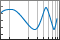Description

example

semilogx(X,Y) plots x- and y-coordinates using a base-10 logarithmic scale on the x-axis and a linear scale on the y-axis.

• To plot a set of coordinates connected by line segments, specify X and Y as vectors of the same length.

• To plot multiple sets of coordinates on the same set of axes, specify at least one of X or Y as a matrix.

example

semilogx(X,Y,LineSpec) creates the plot using the specified line style, marker, and color.

example

semilogx(X1,Y1,...,Xn,Yn) plots multiple pairs of x- and y-coordinates on the same set of axes. Use this syntax as an alternative to specifying coordinates as matrices.

example

semilogx(X1,Y1,LineSpec1,...,Xn,Yn,LineSpecn) assigns specific line styles, markers, and colors to each x-y pair. You can specify LineSpec for some x-y pairs and omit it for others. For example, semilogx(X1,Y1,'o',X2,Y2) specifies markers for the first x-y pair but not the for the second pair.

example

semilogx(Y) plots Y against an implicit set of x-coordinates.

• If Y is a vector, the x-coordinates range from 1 to length(Y).

• If Y is a matrix, the plot contains one line for each column in Y. The x-coordinates range from 1 to the number of rows in Y.

If Y contains complex numbers, semilogx plots the imaginary part of Y versus the real part of Y. However, if you specify both X and Y, MATLAB® ignores the imaginary part.

semilogx(Y,LineSpec) specifies line style, marker, and color.

example

semilogx(___,Name,Value) specifies Line properties using one or more Name,Value pair arguments. The properties apply to all the plotted lines. Specify the Name,Value pairs after all the arguments in any of the previous syntaxes. For a list of properties, see Line Properties.

example

semilogx(ax,___) displays the plot in the target axes. Specify the axes as the first argument in any of the previous syntaxes.

example

lineobj = semilogx(___) returns a Line object or an array of Line objects. Use lineobj to modify properties of the plot after creating it. For a list of properties, see Line Properties.

Examples

collapse all

Define x as a vector of logarithmically spaced values from 0.1 to 100, and define y as a copy of x. Create a linear-log plot of x and y, and call the grid function to show the grid lines.

x = logspace(-1,2);
y = x;
semilogx(x,y)
grid on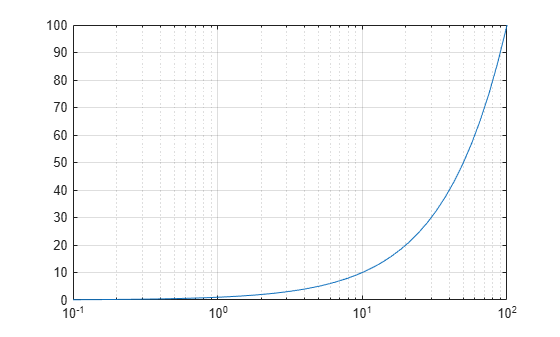Create a vector of logarithmically spaced x-coordinates and two vectors of y-coordinates. Plot two lines by passing comma-separated x-y pairs to semilogx.

x = logspace(-1,2);
y1 = x;
y2 = -x;
semilogx(x,y1,x,y2)
grid on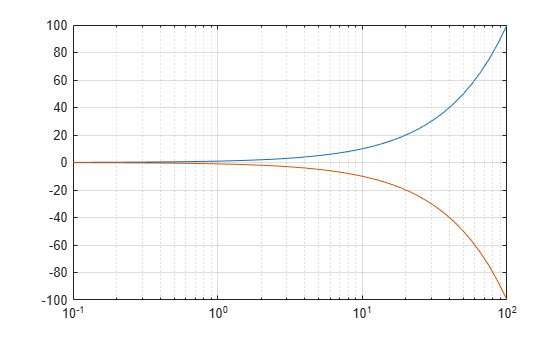Define f as a vector containing the frequencies from 10 Hz to 100,000 Hz. Define gain as a vector of power gain values in decibels. Then plot the gain values against frequency.

f = logspace(1,5,100);
v = linspace(-50,50,100);
gain = (1-exp(5*(2.5*v.^2)./7500))/14;
semilogx(f,gain)
grid on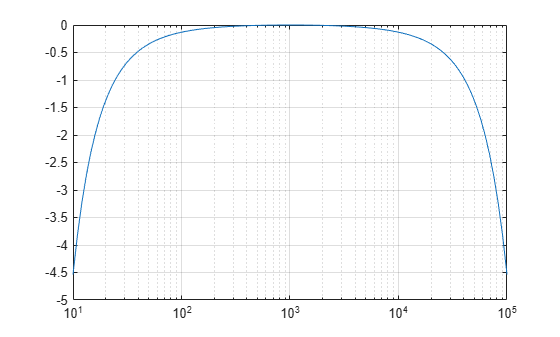Call the yticks function to reposition the y-axis tick values at whole-number increments along the y-axis. Then create x- and y-axis labels by calling the xlabel and ylabel functions.

yticks([-5 -4 -3 -2 -1 0])
xlabel ('Freqency (Hz)')
ylabel('Power Gain (dB)')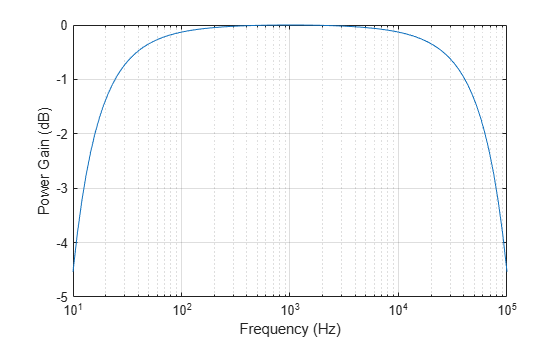Create a set of x- and y-coordinates and display them in a linear-log plot. Specify the line style as 'o' to display circular markers without connecting lines. Specify the marker fill color as the RGB triplet [0 0.447 0.741], which corresponds to a dark shade of blue.

x = logspace(-1,2,15);
y = 12 + x;
semilogx(x,y,'o','MarkerFaceColor',[0 0.447 0.741])
grid on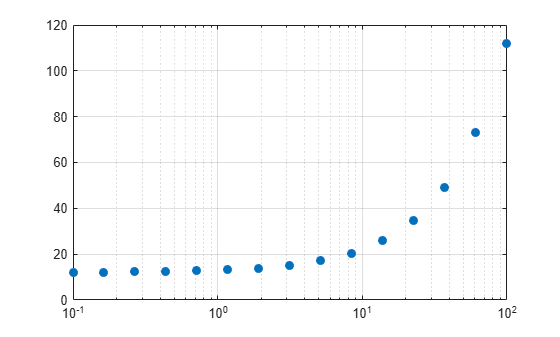Create a vector of logarithmically spaced x-coordinates and two vectors of y-coordinates. Then plot two lines by passing comma-separated x-y pairs to semilogx. Display a legend by calling the legend function.

x = logspace(1,4,100);
v = linspace(-50,50,100);
y1 = 100*exp(-1*((v+5).^2)./200);
y2 = 100*exp(-1*(v.^2)./200);
semilogx(x,y1,x,y2,'--')
legend('Measured','Estimated')
grid on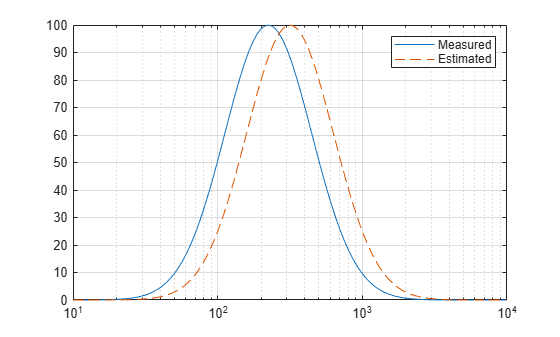When you specify only one coordinate vector, semilogx plots those coordinates against the values 1:length(y). For example, define y as a vector of 5 values between 0 and 40. Create a linear-log plot of y.

y = [0 10 20 30 40];
semilogx(y)
grid on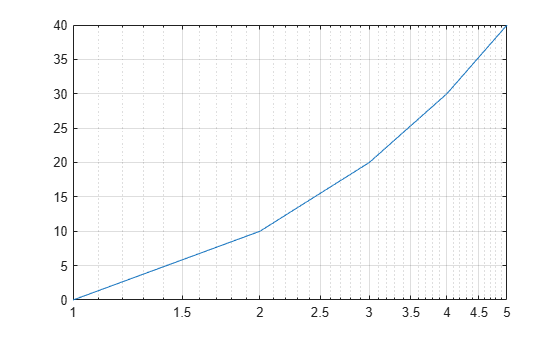If you specify y as a matrix, the columns of y are plotted against the values 1:size(y,1). For example, define y as a 5-by-3 matrix and pass it to the semilogx function. The resulting plot contains 3 lines, each of which has x-coordinates that range from 1 to 5.

y = [ 0    10    20
10    20    30
20    30    40
30    40    50
40    50    60];

semilogx(y)
grid on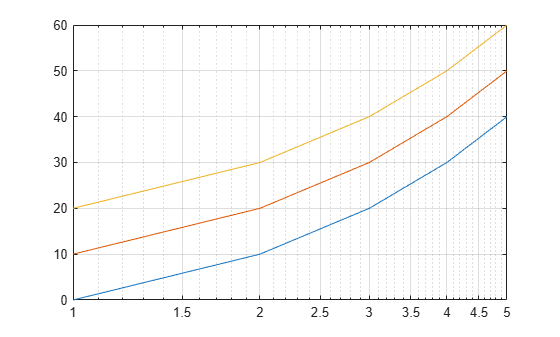Create a tiled chart layout in the 'flow' tile arrangement, so that the axes fill the available space in the layout. Next, call the nexttile function to create an axes object and return it as ax1. Then display a linear-log plot by passing ax1 to the semilogx function.

tiledlayout('flow')
ax1 = nexttile;
x = logspace(-1,2);
y1 = 1./x;
semilogx(ax1,x,y1)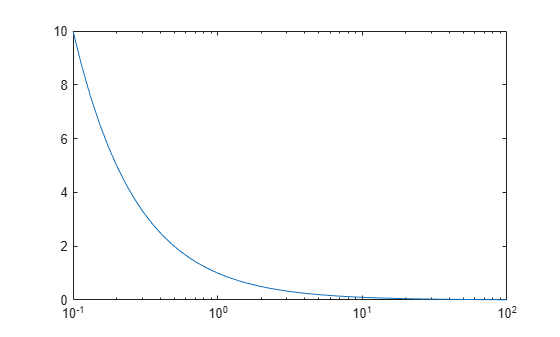Repeat the process to create a second linear-log plot.

ax2 = nexttile;
y2 = x;
semilogx(ax2,x,y2)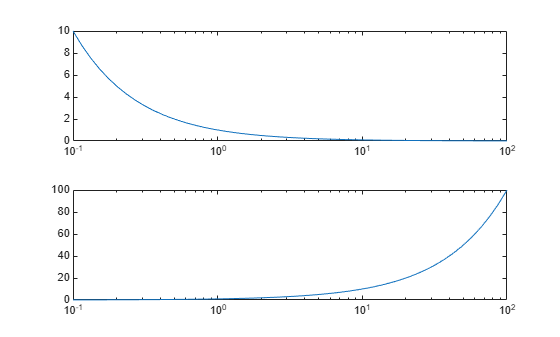Create a linear-log plot containing two lines, and return the line objects in the variable slg.

x = logspace(-1,2);
y1 = x;
y2 = -x;
slg = semilogx(x,y1,x,y2);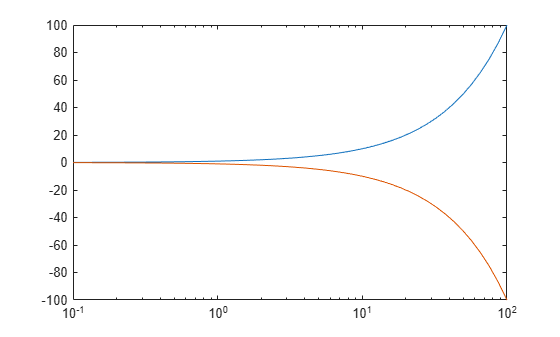Change the width of the first line to 3, and change the color of the second line to purple.

slg(1).LineWidth = 3;
slg(2).Color = [0.4 0 1];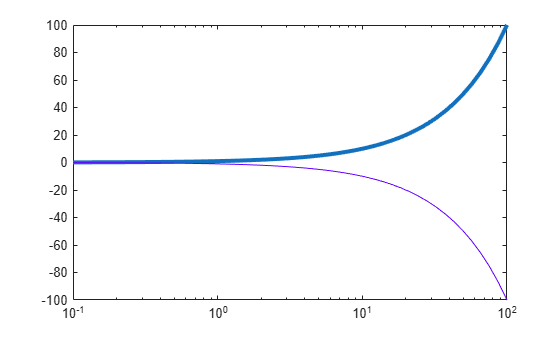Insert NaN values wherever there are discontinuities in your data. The semilogx function displays gaps at those locations.

Create a pair of x- and y-coordinate vectors. Replace the fortieth y-coordinate with a NaN value. Then create a linear-log plot of x and y.

x = logspace(-1,2);
y = x;
y(40) = NaN;
semilogx(x,y)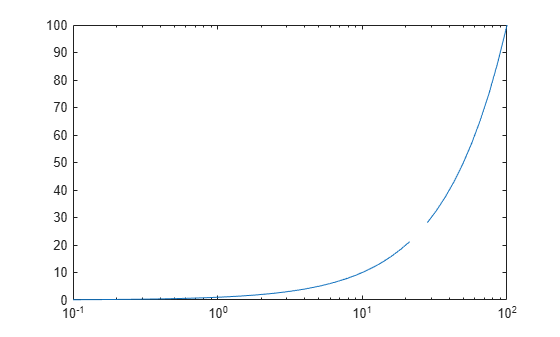Input Arguments

collapse all

Log scale coordinates, specified as a scalar, vector, or matrix. The size and shape of X depends on the shape of your data and the type of plot you want to create. This table describes the most common situations.

Type of PlotHow to Specify Coordinates
Single point

Specify X and Y as scalars and include a marker. For example:

semilogx(1,2,'o')

One set of points

Specify X and Y as any combination of row or column vectors of the same length. For example:

semilogx([1 2 3],[4; 5; 6])

Multiple sets of points
(using vectors)

Specify consecutive pairs of X and Y vectors. For example:

semilogx([1 2 3],[4 5 6],[1 2 3],[7 8 9])

Multiple sets of points
(using matrices)

If all the sets share the same x- or y-coordinates, specify the shared coordinates as a vector and the other coordinates as a matrix. The length of the vector must match one of the dimensions of the matrix. For example:

semilogx([1 2 3],[4 5 6; 7 8 9])
If the matrix is square, semilogx plots one line for each column in the matrix.

Alternatively, specify X and Y as matrices of equal size. In this case, semilogx plots each column of Y against the corresponding column of X. For example:

semilogx([1 2 3; 4 5 6],[7 8 9; 10 11 12])

semilogx might exclude coordinates in some cases:

• If the log scale coordinates include positive and negative values, only the positive values are displayed.

• If the log scale coordinates are all negative, all of the values are displayed on a log scale with the appropriate sign.

• Log scale values of zero are not displayed.

Data Types: single | double | int8 | int16 | int32 | int64 | uint8 | uint16 | uint32 | uint64

Linear scale coordinates, specified as a scalar, vector, or matrix. The size and shape of Y depends on the shape of your data and the type of plot you want to create. This table describes the most common situations.

Type of PlotHow to Specify Coordinates
Single point

Specify X and Y as scalars and include a marker. For example:

semilogx(1,2,'o')

One set of points

Specify X and Y as any combination of row or column vectors of the same length. For example:

semilogx([1 2 3],[4; 5; 6])

Multiple sets of points
(using vectors)

Specify consecutive pairs of X and Y vectors. For example:

semilogx([1 2 3],[4 5 6],[1 2 3],[7 8 9])

Multiple sets of points
(using matrices)

If all the sets share the same x- or y-coordinates, specify the shared coordinates as a vector and the other coordinates as a matrix. The length of the vector must match one of the dimensions of the matrix. For example:

semilogx([1 2 3],[4 5 6; 7 8 9])
If the matrix is square, semilogx plots one line for each column in the matrix.

Alternatively, specify X and Y as matrices of equal size. In this case, semilogx plots each column of Y against the corresponding column of X. For example:

semilogx([1 2 3; 4 5 6],[7 8 9; 10 11 12])

Data Types: single | double | int8 | int16 | int32 | int64 | uint8 | uint16 | uint32 | uint64 | categorical | datetime | duration

Line style, marker, and color, specified as a character vector or string containing symbols. The symbols can appear in any order. You do not need to specify all three characteristics (line style, marker, and color). For example, if you omit the line style and specify the marker, then the plot shows only the marker and no line.

Example: '--or' is a red dashed line with circle markers

Line StyleDescriptionResulting Line
'-'Solid line'--'Dashed line':'Dotted line'-.'Dash-dotted lineMarkerDescriptionResulting Marker
'o'Circle'+'Plus sign'*'Asterisk'.'Point'x'Cross'_'Horizontal line'|'Vertical line's'Square'd'Diamond'^'Upward-pointing triangle'v'Downward-pointing triangle'>'Right-pointing triangle'<'Left-pointing triangle'p'Pentagram'h'HexagramColor NameShort NameRGB TripletAppearance
'red''r'[1 0 0]'green''g'[0 1 0]'blue''b'[0 0 1]'cyan' 'c'[0 1 1]'magenta''m'[1 0 1]'yellow''y'[1 1 0]'black''k'[0 0 0]'white''w'[1 1 1]Target axes, specified as an Axes object. If you do not specify the axes and if the current axes is Cartesian, then semilogx uses the current axes.

Name-Value Arguments

Specify optional comma-separated pairs of Name,Value arguments. Name is the argument name and Value is the corresponding value. Name must appear inside quotes. You can specify several name and value pair arguments in any order as Name1,Value1,...,NameN,ValueN.

Example: semilogx([1 2],[3 4],'Color','red') specifies a red line for the plot.

Note

The properties listed here are only a subset. For a complete list, see Line Properties.

Color, specified as an RGB triplet, a hexadecimal color code, a color name, or a short name. The color you specify sets the line color. It also sets the marker edge color when the MarkerEdgeColor property is set to 'auto'.

For a custom color, specify an RGB triplet or a hexadecimal color code.

• An RGB triplet is a three-element row vector whose elements specify the intensities of the red, green, and blue components of the color. The intensities must be in the range [0,1]; for example, [0.4 0.6 0.7].

• A hexadecimal color code is a character vector or a string scalar that starts with a hash symbol (#) followed by three or six hexadecimal digits, which can range from 0 to F. The values are not case sensitive. Thus, the color codes '#FF8800', '#ff8800', '#F80', and '#f80' are equivalent.

Alternatively, you can specify some common colors by name. This table lists the named color options, the equivalent RGB triplets, and hexadecimal color codes.

Color NameShort NameRGB TripletHexadecimal Color CodeAppearance
'red''r'[1 0 0]'#FF0000''green''g'[0 1 0]'#00FF00''blue''b'[0 0 1]'#0000FF''cyan' 'c'[0 1 1]'#00FFFF''magenta''m'[1 0 1]'#FF00FF''yellow''y'[1 1 0]'#FFFF00''black''k'[0 0 0]'#000000''white''w'[1 1 1]'#FFFFFF''none'Not applicableNot applicableNot applicableNo color

Here are the RGB triplets and hexadecimal color codes for the default colors MATLAB uses in many types of plots.

[0 0.4470 0.7410]'#0072BD'[0.8500 0.3250 0.0980]'#D95319'[0.9290 0.6940 0.1250]'#EDB120'[0.4940 0.1840 0.5560]'#7E2F8E'[0.4660 0.6740 0.1880]'#77AC30'[0.3010 0.7450 0.9330]'#4DBEEE'[0.6350 0.0780 0.1840]'#A2142F'Line width, specified as a positive value in points, where 1 point = 1/72 of an inch. If the line has markers, then the line width also affects the marker edges.

The line width cannot be thinner than the width of a pixel. If you set the line width to a value that is less than the width of a pixel on your system, the line displays as one pixel wide.

Marker size, specified as a positive value in points, where 1 point = 1/72 of an inch.

Marker outline color, specified as 'auto', an RGB triplet, a hexadecimal color code, a color name, or a short name. The default value of 'auto' uses the same color as the Color property.

For a custom color, specify an RGB triplet or a hexadecimal color code.

• An RGB triplet is a three-element row vector whose elements specify the intensities of the red, green, and blue components of the color. The intensities must be in the range [0,1]; for example, [0.4 0.6 0.7].

• A hexadecimal color code is a character vector or a string scalar that starts with a hash symbol (#) followed by three or six hexadecimal digits, which can range from 0 to F. The values are not case sensitive. Thus, the color codes '#FF8800', '#ff8800', '#F80', and '#f80' are equivalent.

Alternatively, you can specify some common colors by name. This table lists the named color options, the equivalent RGB triplets, and hexadecimal color codes.

Color NameShort NameRGB TripletHexadecimal Color CodeAppearance
'red''r'[1 0 0]'#FF0000''green''g'[0 1 0]'#00FF00''blue''b'[0 0 1]'#0000FF''cyan' 'c'[0 1 1]'#00FFFF''magenta''m'[1 0 1]'#FF00FF''yellow''y'[1 1 0]'#FFFF00''black''k'[0 0 0]'#000000''white''w'[1 1 1]'#FFFFFF''none'Not applicableNot applicableNot applicableNo color

Here are the RGB triplets and hexadecimal color codes for the default colors MATLAB uses in many types of plots.

[0 0.4470 0.7410]'#0072BD'[0.8500 0.3250 0.0980]'#D95319'[0.9290 0.6940 0.1250]'#EDB120'[0.4940 0.1840 0.5560]'#7E2F8E'[0.4660 0.6740 0.1880]'#77AC30'[0.3010 0.7450 0.9330]'#4DBEEE'[0.6350 0.0780 0.1840]'#A2142F'Marker fill color, specified as 'auto', an RGB triplet, a hexadecimal color code, a color name, or a short name. The 'auto' option uses the same color as the Color property of the parent axes. If you specify 'auto' and the axes plot box is invisible, the marker fill color is the color of the figure.

For a custom color, specify an RGB triplet or a hexadecimal color code.

• An RGB triplet is a three-element row vector whose elements specify the intensities of the red, green, and blue components of the color. The intensities must be in the range [0,1]; for example, [0.4 0.6 0.7].

• A hexadecimal color code is a character vector or a string scalar that starts with a hash symbol (#) followed by three or six hexadecimal digits, which can range from 0 to F. The values are not case sensitive. Thus, the color codes '#FF8800', '#ff8800', '#F80', and '#f80' are equivalent.

Alternatively, you can specify some common colors by name. This table lists the named color options, the equivalent RGB triplets, and hexadecimal color codes.

Color NameShort NameRGB TripletHexadecimal Color CodeAppearance
'red''r'[1 0 0]'#FF0000''green''g'[0 1 0]'#00FF00''blue''b'[0 0 1]'#0000FF''cyan' 'c'[0 1 1]'#00FFFF''magenta''m'[1 0 1]'#FF00FF''yellow''y'[1 1 0]'#FFFF00''black''k'[0 0 0]'#000000''white''w'[1 1 1]'#FFFFFF''none'Not applicableNot applicableNot applicableNo color

Here are the RGB triplets and hexadecimal color codes for the default colors MATLAB uses in many types of plots.

[0 0.4470 0.7410]'#0072BD'[0.8500 0.3250 0.0980]'#D95319'[0.9290 0.6940 0.1250]'#EDB120'[0.4940 0.1840 0.5560]'#7E2F8E'[0.4660 0.6740 0.1880]'#77AC30'[0.3010 0.7450 0.9330]'#4DBEEE'[0.6350 0.0780 0.1840]'#A2142F'Tips

• The semilogx function uses colors and line styles based on the ColorOrder and LineStyleOrder properties of the axes. semilogx cycles through the colors with the first line style. Then, it cycles through the colors again with each additional line style.

You can change the colors and the line styles after plotting by setting the ColorOrder or LineStyleOrder properties on the axes. You can also call the colororder function to change the color order for all the axes in the figure.

Algorithms

The semilogx function plots x-coordinates on a log scale by setting the XScale property of the axes to 'log'. However, if the axes hold state is 'on' before you call semilogx, the property does not change, and the x-coordinates might display on a linear scale.